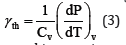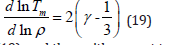• Submissions# Analysis of the Density Dependence of the Grüneisen Parameter for Materials at High Pressures

Dwivedi A*

Department of Physics, Institute of Basic Sciences, Khandari Campus, India

*Corresponding author: Dwivedi A, Department of Physics, Institute of Basic Sciences, Khandari Campus, Agra - 282 002, India

Submission: August 13, 2022;Published: September 06, 2022

DOI: 10.31031/RDMS.2022.17.000921ISSN: 2576-8840
Volume 17 Issue 5

#### Abstract

Ab initio calculations based on the density functional theory have been used to demonstrate that the Debye frequency is a linear function of density to a high accuracy for several solids under high pressures (U.C. Roy, S.K. Sarkar, Computational Condensed Matter 27 (2021) e 00552). This result has been used in the present study to demonstrate further that the Grüneisen parameter and its high order density derivatives satisfy the thermodynamic constraints for solids at high pressures in the limit of extreme compression.

Keywords: Debye frequency; Grüneisen parameter; Density; Derivatives of gamma; Infinite pressure behaviour

#### Introduction

The Grüneisen parameter γ is of central importance for investing the thermal and elastic properties of materials [1,2]. The Grüneisen parameter is a measure of anharmonicity in the vibrational properties of a crystal. There are two versions of γ, the vibrational Grüneisen parameter γ vibrational and γ thermal [3,4]. The vibrational Grüneisen parameter ,()vibjγfor a particular mode labelled by j is defined as follows .where jω is the vibrational frequency of the jth mode and ρ represents density. In the Debye model, the Debye frequency ωD is used in place of ωj, and we have the following relationship from Eq. (1)where γvib can now be written as γD.

The thermal or thermodynamic Grüneisen parameter γth is defined as followswhere P and T denote the pressure and temperature, respectively. Cv is the ratio of the constant-volume specific heat to the volume of the material. Roy  have performed the vibrational spectrum first-principles electronic structure-based calculations using the Density Functional Theory (DFT)  as implemented in the Quantum Expresso package. These ab initio calculations reveal that the Debye frequency has a linear dependence on density to a high level of accuracy. We can writewhere a and b are material - dependent constants. Using Eqs. (2) and (4) we getEquation (5) can be rewritten as followsAccording to Eq. (6), the ratio of density and gamma depends linearly on density. The second order Grüneisen parameter q is defined as [1,2]Then, Eqs. (5) and (7) yieldIt should be emphasized that at extreme compression, ρ tends to infinity, and therefore, q tends to zero, and γ tends to one.This is an important result according to which the third order Grüneisen parameter is equal to the first order Grüneisen parameter. Thus λ00 and λ. Eq. (6) at initial or reference values of pressure and temperature givesThe density ρ tends to infinity in the limit of extreme compression, Eq. (18) givesγ =1 .

Eq. (18) is useful for determining an analytical formula using the Lindemann law [8,9]Using Eq. (18) in Eq. (19), and then with respect to density, we obtainValues of melting temperature at high pressure can be calculated with the help of Eq. (20) provided pressures corresponding to densities are known using an accurate equation of state such as the Stacey reciprocal K-primed equation .

The results for γ , q and λ obtained in the present study are consistent with the thermodynamic constraints for solids in the limit of infinite pressure reported by Stacey . According to Stacey we have γ>2/3, q0 → 0, and λ∞ remains positive and finite. It has been found by Shanker et al.  that λ must be less than K' , the pressure derivative of bulk modulus in the limit of infinite pressure. The result λ=1 obtained in the present study is consistent with the constraint λ < K' because K' is greater than 5/3 as derived by Stacey  on the basis of fundamental thermodynamics of solids.

1. Anderson OL (1995) Equation of state of solids for geophysics and ceramic science. Oxford University Press, New York, USA.
2. Stacey FD, Davis PM (2004) High pressure equations of state with applications to the lower mantle and core. Phys Earth Planet Inter 142(3-4): 137-184.
3. Vocadlo L, Poirer JP, Price GD (2000) Grüneisen parameters and isothermal equations of state. Am Mineral 85(2): 390-395.
4. Anderson OL (2000) The Grüneisen ratio for the last 30 years. Geophys J Int 143(2): 279-294.
5. Ashcroft N, Mermin N (1976) Solid State Physics, HRW International Editions, Holt, Rinchart and Winston.
6. Roy UC, Sarkar SK (2021) Ab initio study of the density dependence of the Grϋneisen parameter at pressures up to 360 GPa. Comput Condens Matter 27: e00552.
7. Martin RM (2004) Electronic structure, basic theory and practical methods. Cambridge University Press, Cambridge, UK.
8. Lindemann FA (1910) Phys Z 11:
9. Gilvarry JJ (1956) The Lindemann and Grüneisen Laws. Phys Rev 102:
10. Stacey FD (2000) The K‐primed approach to high‐pressure equations of state. Geophysics J Int 143(3): 621-628.
11. Shanker J, Sunil K, Sharma BS (2017) The Grüneisen parameter and its higher order derivatives for the Earth lower mantle and core. Physics of the Earth and Planetary Interiors 262: 41-47.

We at Crimson Publishing are a group of people with a combined passion for science and research, who wants to bring to the world a unified platform where all scientific know-how is available read more...